# 静态贝叶斯网络

Author: Steven Date: May 2, 2016 Updated On: May 12, 2020
Categories: 概率图模型
5.8k words in total, 21 minutes required.

## 1. 贝叶斯定理

### 1.1 频率主义派和贝叶斯派的思考

• 频率主义（Frequentist）派： 需要推断的参数$\theta$是一个未知常数；同时，样本是随机的，因此重点研究对象为样本空间，大部分概率计算都是针对样本集$\chi$的分布进行的
• 贝叶斯（Bayesian）派： 参数$\theta$是未观测的随机变量，其本身也具有一定的分布。而样本$\chi$是固定的，由于样本是固定的，需要重点研究的对象为参数$\theta$的分布（假定参数服从一个先验分布，然后基于观测到的数据来计算参数的后验分布

$\pi(\theta) + \chi \implies \pi(\theta \mid x)$

### 1.2 贝叶斯公式

$P(A \cap B) = P(B \mid A)P(A) = P(A \mid B)P(B)$

$P(A \mid B) = \frac{P(B \mid A)P(A)}{P(B)}$

$P(A_i \mid B) = \frac{P(B \mid A_i)P(A_i)}{P(B)} = \frac{P(B \mid A_i)P(A_i)}{\sum_{j}P(B \mid A_j)P(A_j)}$

中还给出了一个利用贝叶斯原理，进行搜索拼写检查的实例。

## 2. 贝叶斯网络模型

• 一个贝叶斯网络是一个有向无环图（Directed Acyclic Graph, DAG），由代表变量结点及连接这些结点的有向边构成。
• 结点代表随机变量，结点间的有向边代表了结点间的互相关系（由父结点指向其后代结点），即条件依赖（conditional dependencies），用条件概率进行表达关系强度，没有父结点的用先验概率进行信息表达。
• 结点变量可以是任何问题的抽象，如：测试值，观测现象，意见征询等。适用于表达和分析不确定性和概率性的事件，应用于有条件地依赖多种控制因素的决策，可以从不完全、不精确或不确定的知识或信息中做出推断。
• 因果关系不能循环，即结果不能推回原因。
• 因此推断是图中的一条路径
• 朴素贝叶斯、马尔可夫链和隐马尔可夫模型都是贝叶斯网络的几种特例。

### 2.1 贝叶斯网络与马尔科夫链的比较

• 马尔科夫链描述的是状态序列，很多时候事物之间的相互关系并不能用一条链串起来，比如研究疾病和成因之间的关系便是错综复杂的。这个时候就要用到贝叶斯网络：每个状态只跟与之直接相连的状态有关，而跟与它间接相连的状态没直接关系。但是只要在这个有向图上，有通路连接两个状态，就说明这两个状态是有关的，可能是间接相关。状态之间弧用转移概率来表示，构成了信念网络。

• 叶斯网络的拓扑结构比马尔可夫链灵活，不受马尔科夫链的链状结构的约束，更准确的描述事件之间的相关性。马尔科夫链是贝叶斯网络的一个特例，而贝叶斯网络是马尔科夫链的推广。

• 拓扑结构和状态之间的相关概率，对应结构训练和参数训练。贝叶斯网络的训练比较复杂，从理论上讲是一个NP完备问题，对于现在计算机是不可计算的，但对于某些具体应用可以进行简化并在计算机上实现。

### 2.2 贝叶斯网络与神经网络的比较

• 贝叶斯是生成模型（联合概率），神经网络是判别模型（已知模型，训练参数）
• 人工神经网络在结构上是完全标准化的，而贝叶斯网络更灵活
• 虽然神经元函数为非线性函数，但是各个变量只能先进行线性组合，最后对一个变量（即前面组合出来的结果）进行非线性变换，因此用计算机实现起来比较容易。而在贝叶斯网络中，变量可以组合成任意的函数，毫无限制，在获得灵活性的同时，也增加了复杂性
• 贝叶斯网络更容易上下文前后的相关性，因此可以解码一个输入的序列，比如将一段语音识别成文字，或者将一个英语句子翻译成中文。而人工神经网络的输出是独立，它可以识别一个个字，但很难处理一个序列，因此它主要的应用常常是估计一个概率模型的参数，比如语音识别中声学模型参数的训练、机器翻译中语音模型参数的训练等等，而不是作为解码器
• 贝叶斯网络是白盒，神经网络是黑盒

• 都是有向图，每一个结点的取值只取决于前一级的结点，而与更前面的结点无关，即遵从马尔科夫假设
• 训练方式相似，都是统计模型
• 训练过程的计算量都特别的大

## 3. 贝叶斯网络中的独立性

### 3.1 图的结构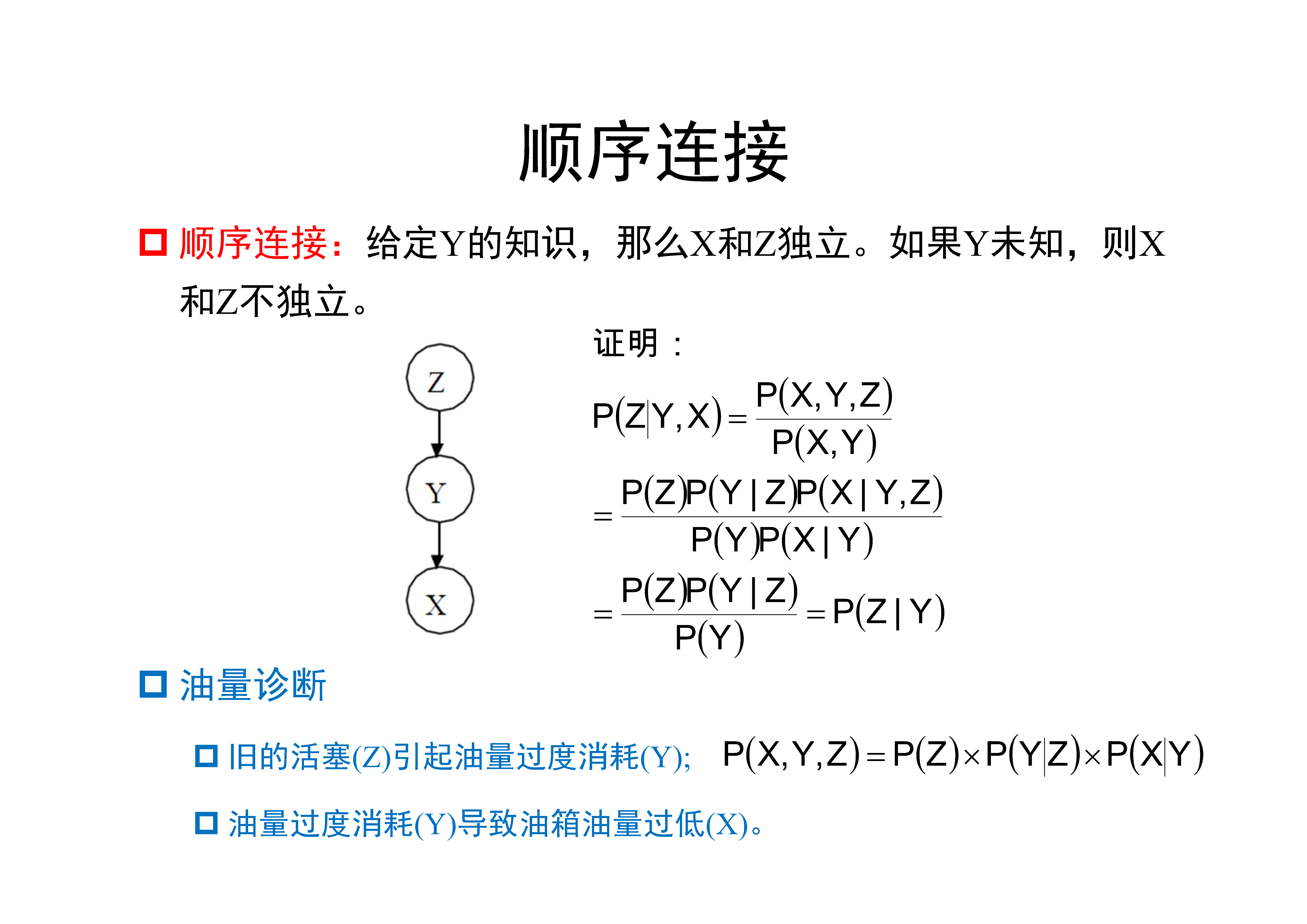顺序（Serial）连接 head-to-tail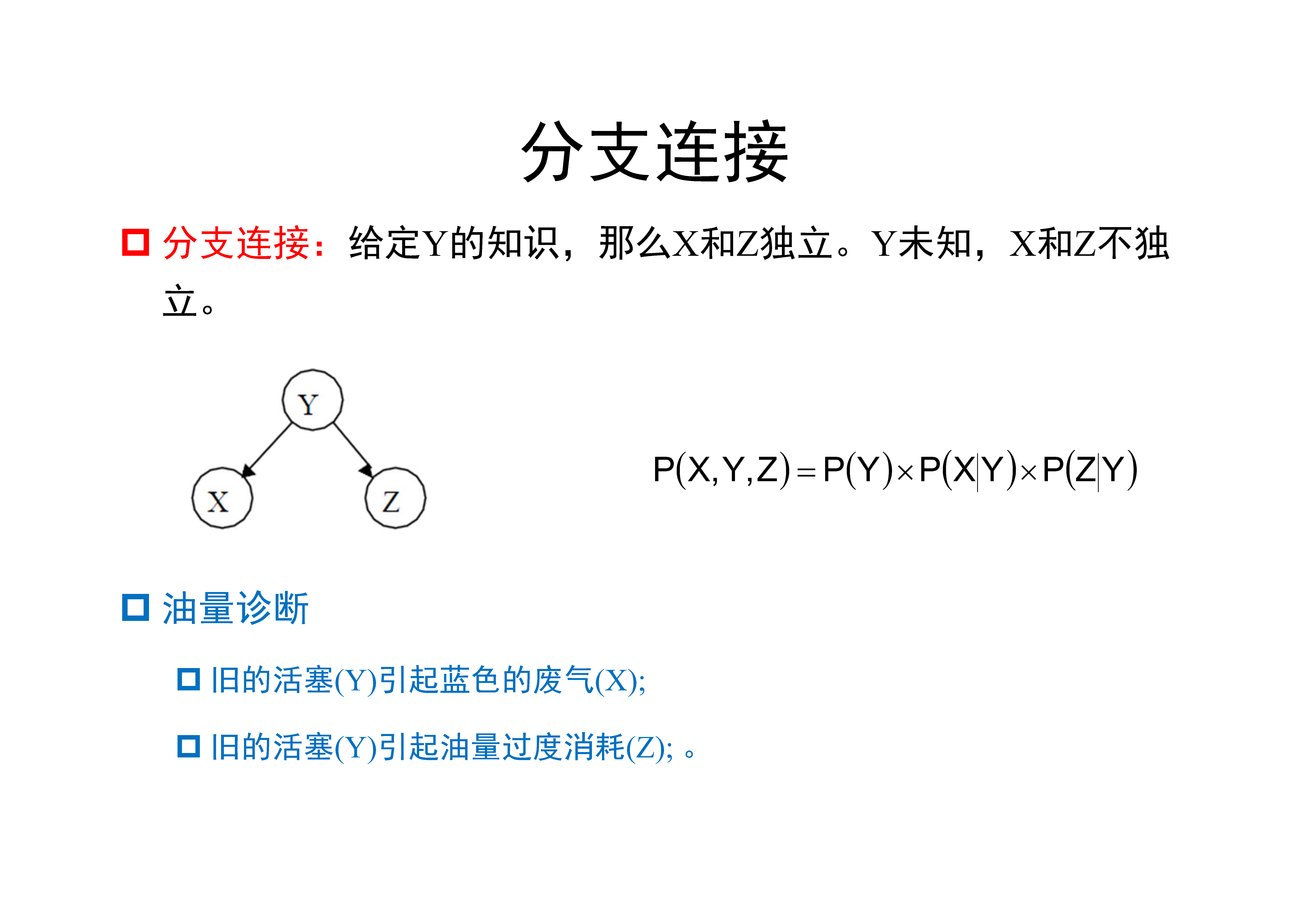分支（Diverging）连接 tail-to-tail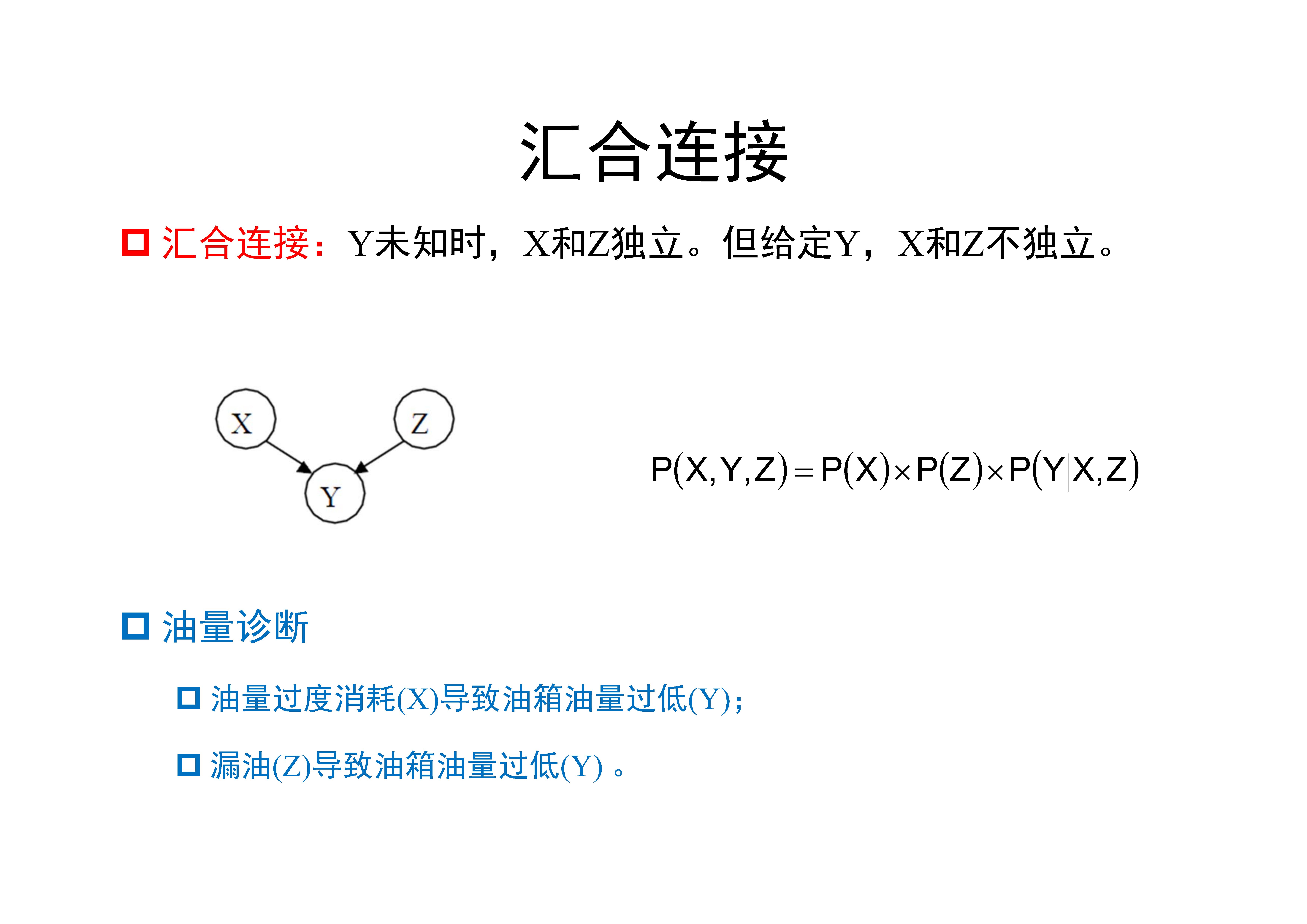汇合（Converging）连接 head-to-head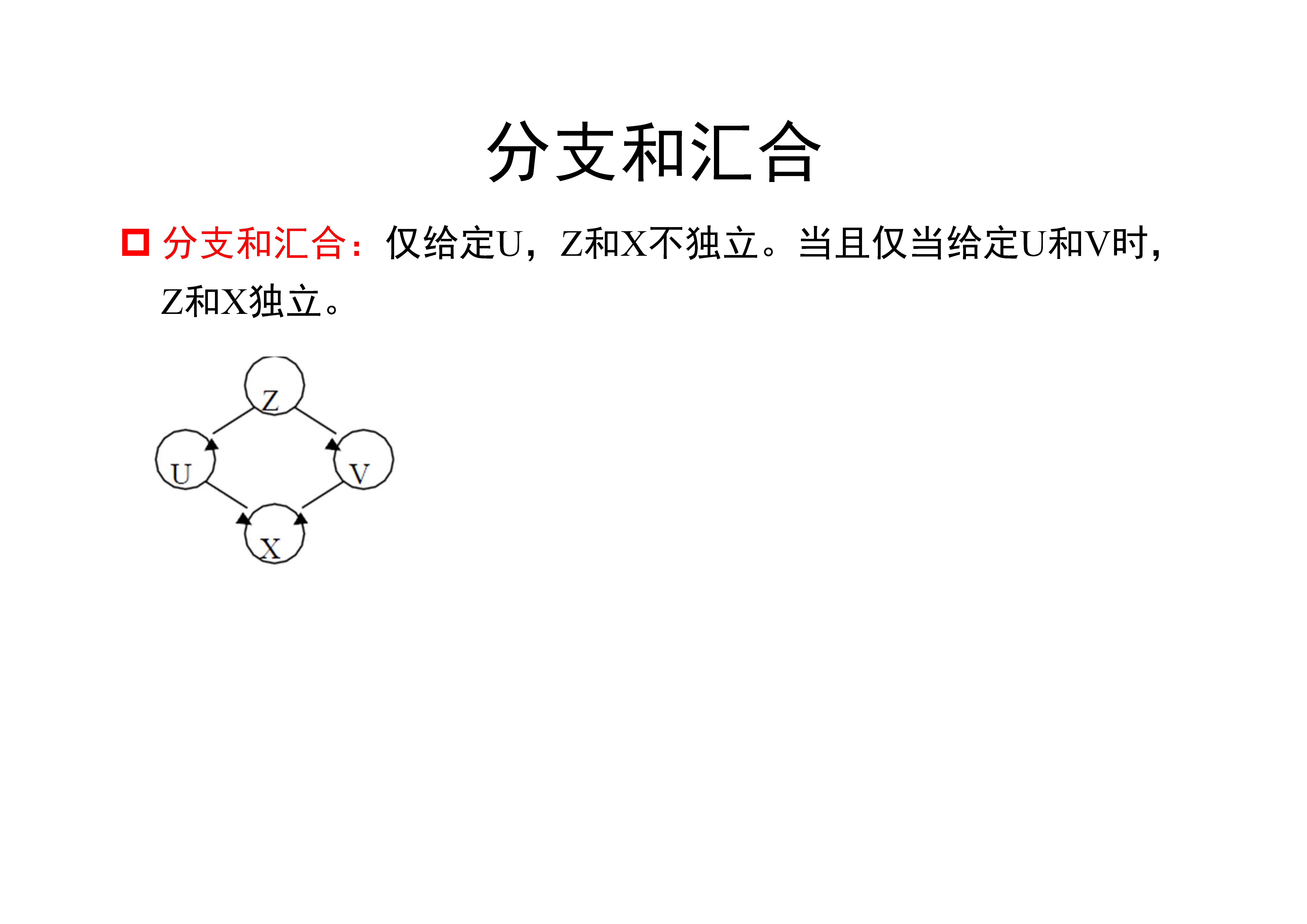分支和汇合

### 3.2 $d$-可分（$d$-separation）

$A$和$B$被一组随机变量$E$ $d$-可分，当且仅当它们间的所有路径都是堵塞的。

1. 连接是顺序或者分支的，$V$在$E$中。
2. 连接是汇合的，则$V$和它的子结点都不在$E$中。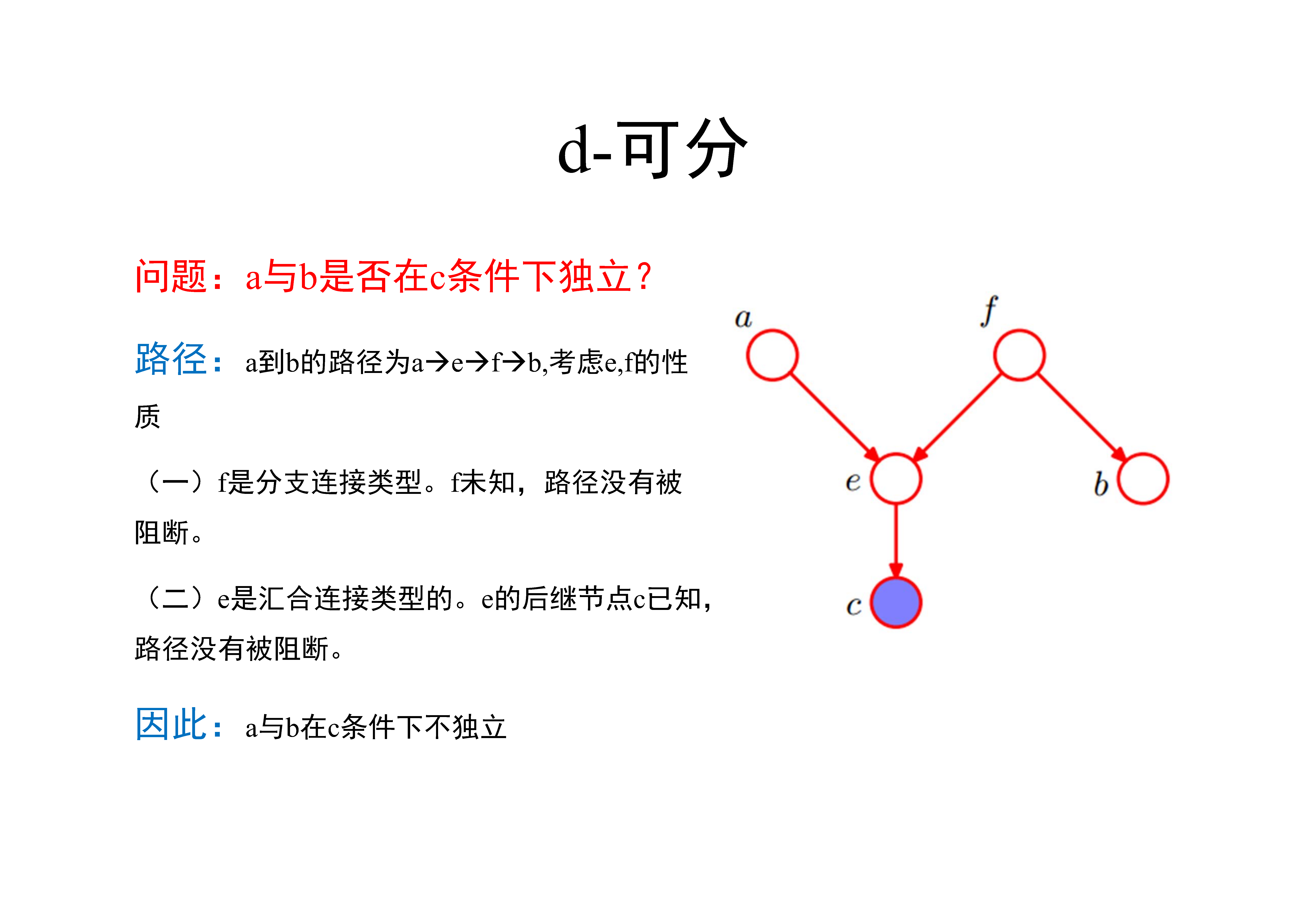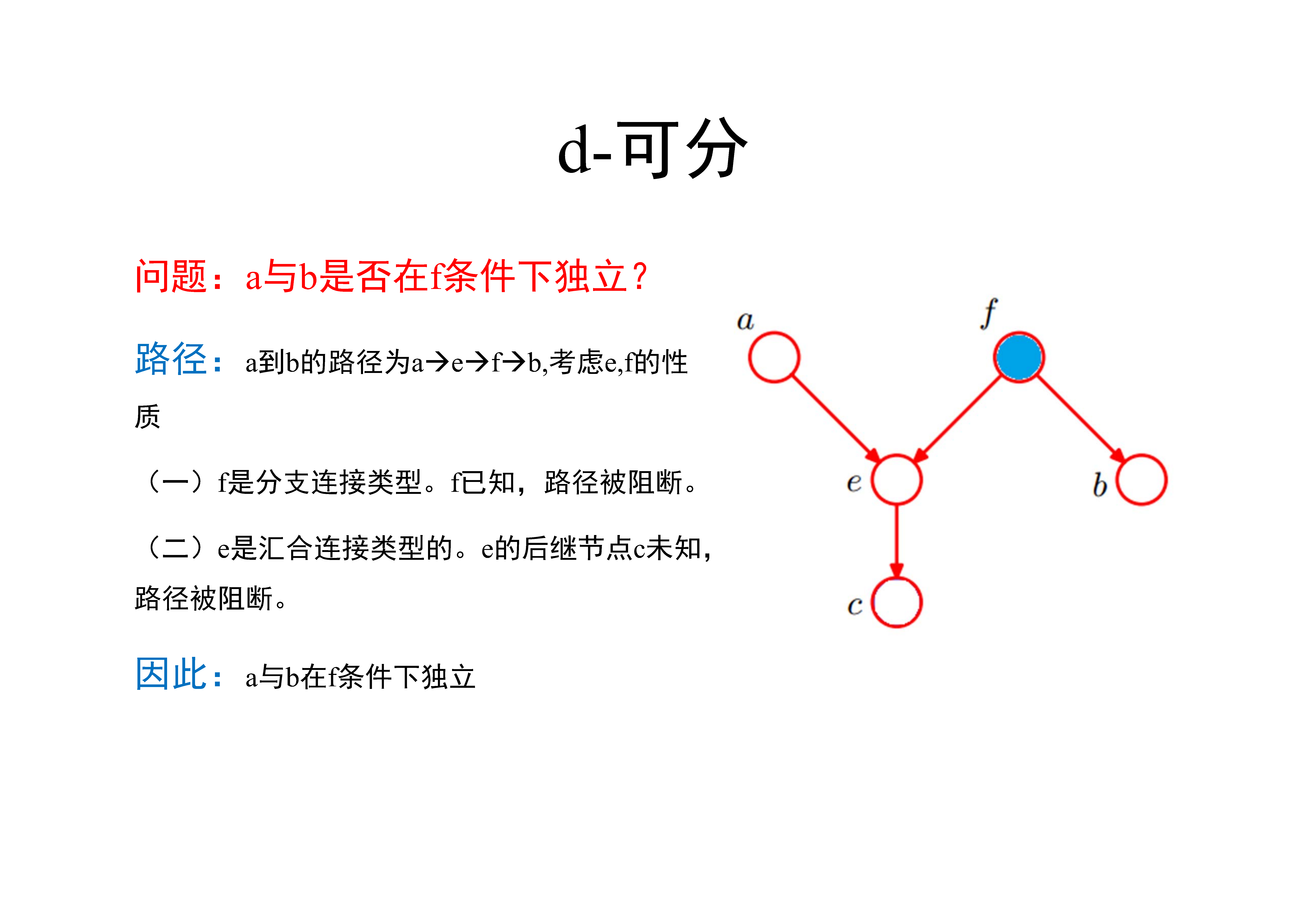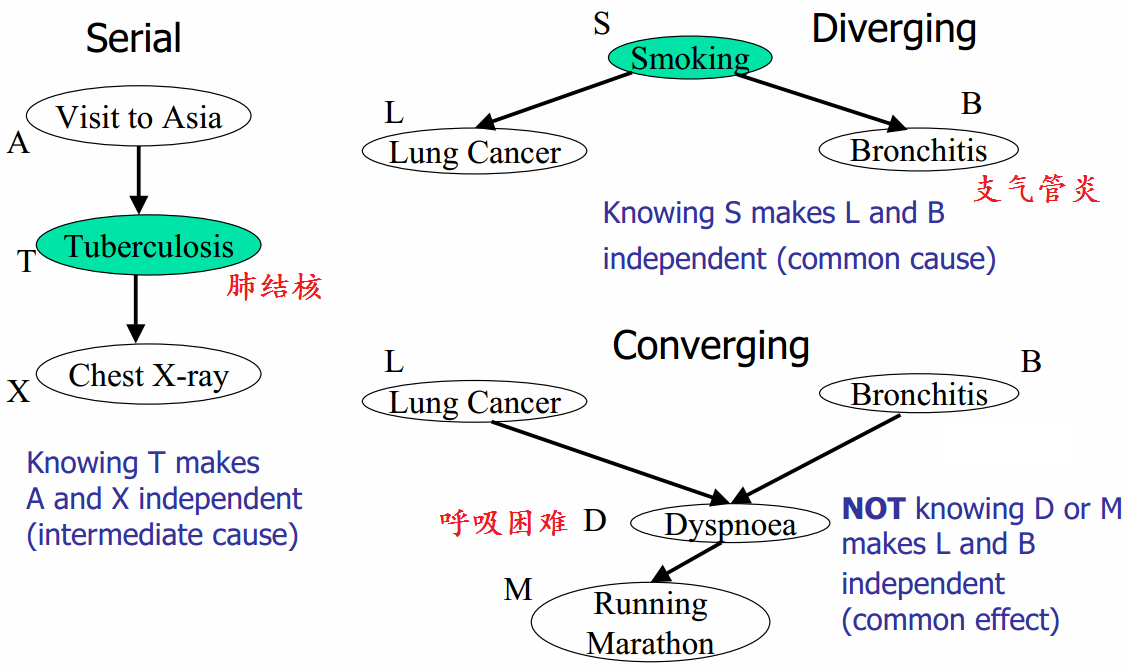左边部分是head-to-tail，给定T时，A和X独立；右上角是tail-to-tail，给定S时，L和B独立；右下角是head-to-head，未给定D和/或M时，L和B独立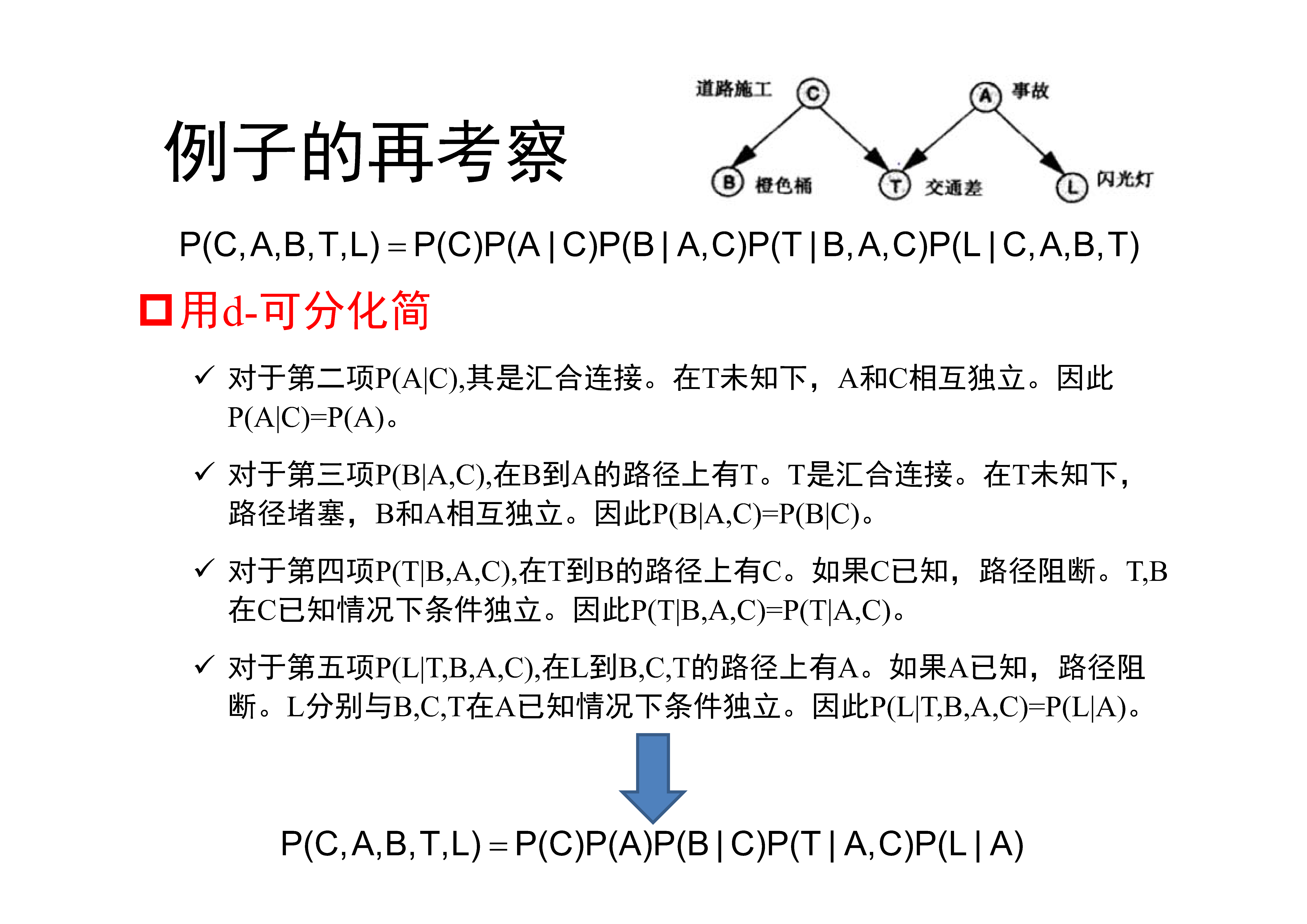一个公式化简的例子

## 4. 贝叶斯网络的结构

• 确定随机变量间的拓扑关系，形成DAG。这一步通常需要领域专家完成，而想要建立一个好的拓扑结构，通常需要不断迭代和改进才可以，需要用到机器学习得到。
• 训练贝叶斯网络。即完成条件概率表的构造，如果每个随机变量的值都是可以直接观察的，那么这一步的训练是直观的，方法类似于朴素贝叶斯分类。但是通常贝叶斯网络的中存在隐藏变量结点，那么训练方法就是比较复杂，例如使用梯度下降法。

### 4.1 贝叶斯网络的构建

• 定义变量
• 在领域知识下选择合适变量，或选择重要因子
• 结构学习
• 构建有向无环图
• 能够很好地解释数据，反映变量间的依赖关系或者独立性
• 不造成过拟合
• 参数学习
• 学习结点的分布参数，即每条边对应的条件概率分布

1. 选择一组刻画问题的随机变量$\{ X_1, X_2, \ldots, X_n \}$
2. 确定一个变量顺序$a = \langle X_1, X_2, \ldots, X_n \rangle$
3. 参数学习从一个空图出发，按照顺序$a$逐个将变量加入$\xi$中
4. 假设当前加入的变量是$X_i$，此时$\xi$中已经包含变量$X_1, \ldots, X_{i-1}$
• 在$X_1, \ldots, X_{i-1}$中选择一个尽可能小的子集$\pi(X_i)$，使得假设“给定$\pi(X_i)$，$X_i$与$\xi$中其他变量条件独立”合理
• 从$\pi(X_i)$中每一个结点添加一条到$X_i$的有向边

### 4.2 贝叶斯网络的结构学习

• 利用训练样本集，尽可能结合先验知识，确定和训练样本集合匹配最好的贝叶斯网络结构
• 对于$n$个变量，可能的结构数目有以下关系 $f(n) = \sum_{i=1}^n (-1)^{i+1} \frac{n!}{i!(n-i)!} 2^{2(n-1)} f(n-i)$
• 结构学习是一个典型的NP-Hard问题

#### 4.2.1 基于评分和搜索的方法

• 利用评分函数（score function），寻找与训练样本匹配最好（且结构最优）的贝叶斯网络结构：

$G^{\star} = \arg\max\limits_{G \subseteq \xi} g(G:D)$

• 评分函数

• 1992年由Cooper and Herskovits首先提出K2评分函数，假设观测到的数据是完备的，且符合多项式分布
• 基于K2评分，Heckerman等人在1995年提出了BD评分函数，假设观测数据服从Dirichlet分布

#### 4.2.2 评分函数的信息论准则

$s(B \mid \mathbf{D}) = f(\theta) \mid B \mid + (- LL(B \mid \mathbf{D}) )$

$LL(B \mid \mathbf{D}) = \sum\limits_{\mathbf{x_i} \in \mathbf{D}} \log P_B(\mathbf{x_i})$

• 若$f(\theta) = 1$，每个参数用1个字节描述，则得到AIC（Akaike Information Criterion）评分函数

$\text{AIC}(B \mid \mathbf{D}) = \mid B \mid + (- LL(B \mid \mathbf{D}) )$

• 若$f(\theta) = \frac{1}{2} \log \mid \mathbf{D} \mid$，则得到BIC（Bayesian Information Criterion）评分函数

$\text{BIC}(B \mid \mathbf{D}) = \frac{1}{2} \log \mid \mathbf{D} \mid \cdot \mid B \mid + (- LL(B \mid \mathbf{D}) )$

• 若$f(\theta) = 0$，则得评分函数退化为负对数似然，学习任务直接退化为极大似然估计

#### 4.2.3 搜索策略

• 搜索策略

• 贪婪搜索、模拟退火、禁忌搜索、遗传算法 等
• 贪婪算法

• 从一个特定网络出发（如一个没有任何连接边的网络）
• 利用搜索算法对网络进行操作（增加/删除边、反转边的方向）
• 根据评分函数对网络进行评分
• 检查新的网络结构是否优于旧的，如是，则继续
• 除贪婪算法外，还可以通过给网络结构施加约束来削减搜索空间

• 例如，将网络结构限定为树形结构等

## 5. 贝叶斯网络的推断

• 因果推断（causal inference）自顶向下
• 由原因推出结论，即根据一定原因，推断出在该原因下结果发生的概率
• 诊断推断（diagnostic inference）自底向上
• 由结论推出原因，即根据产生结果，利用贝叶斯网推断算法，得出导致结果的原因的概率
• 支持推断
• 对发生的现象提供解释，目的是分析原因间的相互影响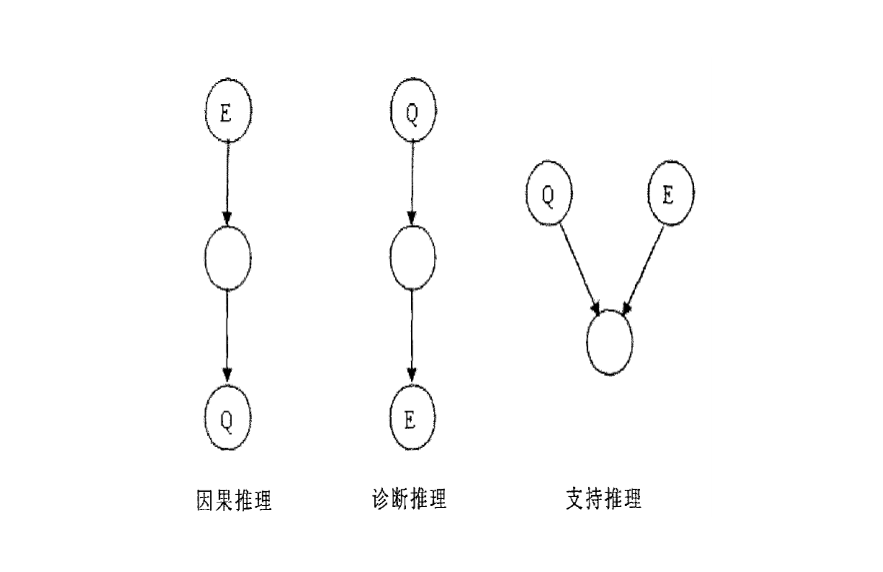贝叶斯网络推断分类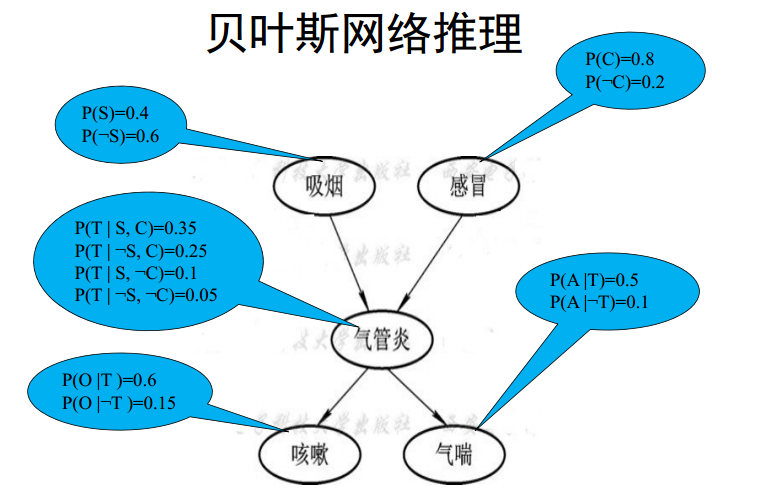一个实例

### 5.1 精确推断

#### 5.1.1 因果推断

• 已知网络中的祖先结点而计算后代结点的条件概率
• 假设已知某人吸烟$(S)$，计算其患气管炎$(T)$的概率$P(T \mid S)$
• 由于$T$还有另一个因结点感冒$(C)$，对概率$P(T \mid S)$进行扩展

$P(T \mid S) = P(T, C \mid S) + P(T, \lnot C \mid S)$

• 对第一项$P(T, C \mid S)$进行扩展，如下

$P(T, C \mid S)$
$=$ $P(T,C,S)/P(S)$
$=$ $P(T \mid C,S)P(C,S)/P(S)$
$=$ $P(T \mid C,S)P(C \mid S)$
$=$ $P(T \mid C,S)P(C)$ //由于$C$和$S$条件独立

• 同理，第二项$P(T, \lnot C \mid S)$可以扩展为$P(T \mid \lnot C,S)P(\lnot C)$。带入原公式，可以得到

$P(T \mid S) = P(T, C \mid S) + P(T, \lnot C \mid S)$ = $P(T \mid C,S)P(C)+P(T \mid \lnot C,S)P(\lnot C)$

• 因此，在上例中，吸烟$S$引起气管炎$T$的概率可以计算为

$P(T \mid S) = 0.35 \cdot 0.8 + 0.1 \cdot 0.2 = 0.3$

• 因果推断解题方法

1. 对于所求的询问结点的条件概率，用所给证据结点和询问结点的所有因结点的联合概率进行重新表达。
2. 对所得表达式进行适当变形, 直到其中的所有概率值都可以从问题贝叶斯网络的条件概率表（CPT, Conditional Probability Table）中得到。
3. 将相关概率值代入概率表达式进行计算即得所求询问结点的条件概率。

#### 5.1.2 诊断推断

• 已知网络中的后代结点而计算祖先结点的条件概率
• 假设已知某人患了气管炎$(T)$，计算其吸烟$(S)$的概率$P(S \mid T)$

$P(S \mid T) = \frac{P(T \mid S)P(S)}{P(T)}$

• 根据上述因果推断，可以得到$P(T \mid S) = 0.3$。由于条件概率表中$P(S) = 0.4$，可得

$P(S \mid T) = \frac{0.3 \cdot 0.4}{P(T)} = \frac{0.12}{P(T)}$

• 根据因果推断，我们还需要得到$P(T \mid \lnot S)$，如下

$P(T \mid \lnot S)$
$= P(T,C \mid \lnot S) + P(T, \lnot C \mid \lnot S)$
$= P(T \mid C, \lnot S)P(C) + P(T \mid \lnot C, \lnot S)P(\lnot C)$
$= 0.25 \cdot 0.8 + 0.05 \cdot 0.2 = 0.21$

• 根据以上和$P(\lnot S) = 0.6$，可以有

$P(\lnot S \mid T) = \frac{0.21 \cdot 0.6}{P(T)} = \frac{0.126}{P(T)}$

• 由于存在关系$P(S \mid T) + P(\lnot S \mid T) = 1$，可以有

$\frac{0.12}{P(T)} + \frac{0.126}{P(T)} = 1 \implies P(T) = 0.246$

• 则，可以进一步得到$P(S \mid T)$

$P(S \mid T) = \frac{0.12}{P(T)} = \frac{0.12}{0.246} = 0.4878$

• 诊断推断解题方法

1. 利用贝叶斯公式将诊断推断问题转化为因果推断问题
2. 利用因果推断结果，导出诊断推断的结果

### 5.2 近似推断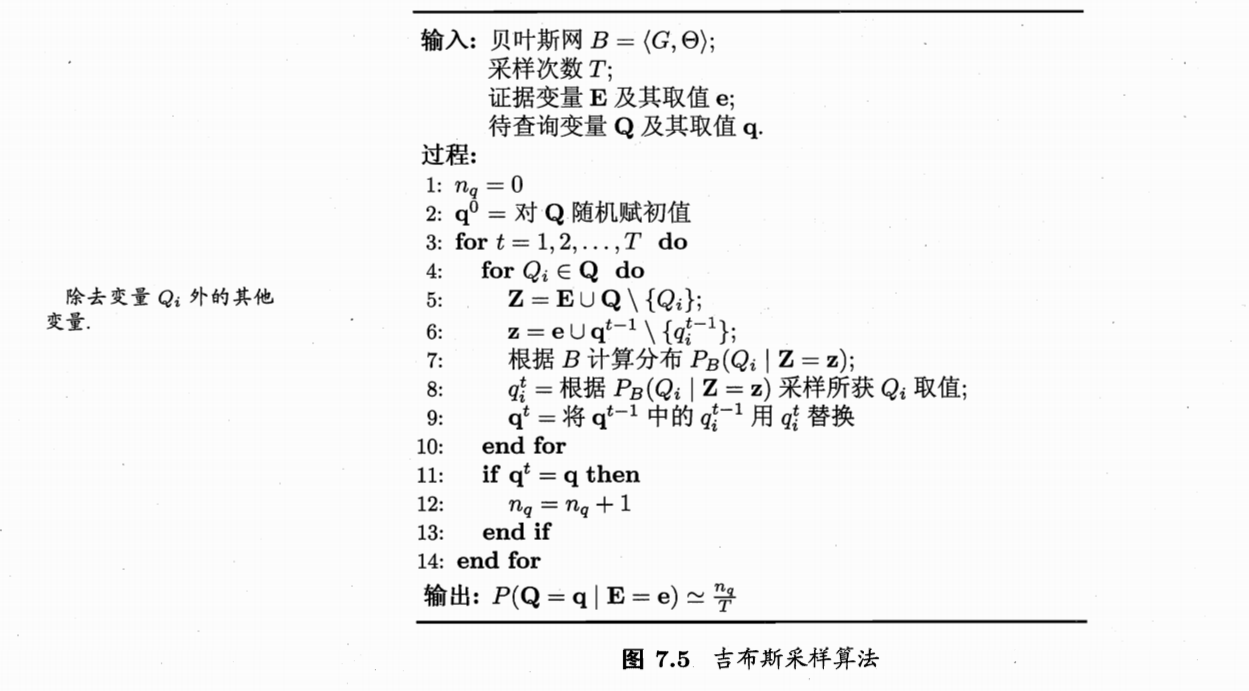吉布斯采样算法

• 行2，随机产生一个对应于证据$\mathbf{E} = \mathbf{e}$的样本$\mathbf{q}^{0}$
• 行3-14，从当前的样本出发，经过$T$次采样，看一共能够得到多少和$\mathbf{q}$一致的采样样本，保存在变量$n_q$中
• 每一步从上一个样本出发，即$\mathbf{q}^{t}$首先取值为$\mathbf{q}^{t-1}$
• 如行4-6所示，对非证据变量$\mathbf{Z}$进行逐一采样，改变其取值
• 如行7所示，取值改变应该参照其采样概率，采样概率可以通过贝叶斯网络$B$和当前其他变量的当前取值$\mathbf{Z} = \mathbf{z}$来获得
• 如行11-13，当采样得到的$\mathbf{q}^{t}$等同于$\mathbf{q}$时，$n_q$计数加1。意味着，我们可以近似地估算出后验概率

$P(\mathbf{Q} = \mathbf{q} \mid \mathbf{E} = \mathbf{e}) \simeq \frac{n_q}{T}$

### 引用

1. 1.
2. 2.
3. 3.
4. 4.
5. 5.
6. 6.《机器学习》，周志华著，清华大学出版社.
7. 7.